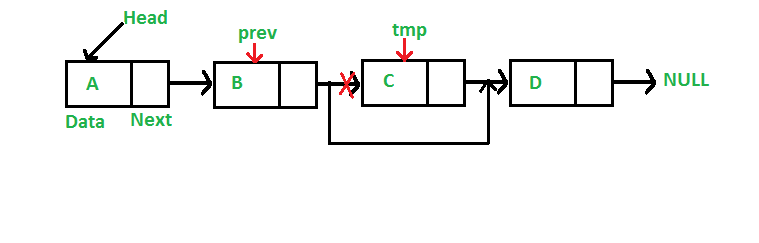# C Program For Deleting A Node In A Linked List

• Last Updated : 26 Dec, 2021

We have discussed Linked List Introduction and Linked List Insertion in previous posts on a singly linked list.
Let us formulate the problem statement to understand the deletion process. Given a ‘key’, delete the first occurrence of this key in the linked list

Iterative Method:
To delete a node from the linked list, we need to do the following steps.
1) Find the previous node of the node to be deleted.
2) Change the next of the previous node.
3) Free memory for the node to be deleted.Since every node of the linked list is dynamically allocated using malloc() in C, we need to call free() for freeing memory allocated for the node to be deleted.

## C

 `// A complete working C program``// to demonstrate deletion in``// singly linked list``#include ``#include `` ` `// A linked list node``struct` `Node {``    ``int` `data;``    ``struct` `Node* next;``};`` ` `/* Given a reference (pointer to pointer) to the head of a``   ``list and an int, inserts a new node on the front of the``   ``list. */``void` `push(``struct` `Node** head_ref, ``int` `new_data)``{``    ``struct` `Node* new_node``        ``= (``struct` `Node*)``malloc``(``sizeof``(``struct` `Node));``    ``new_node->data = new_data;``    ``new_node->next = (*head_ref);``    ``(*head_ref) = new_node;``}`` ` `/* Given a reference (pointer to pointer) to the head of a``   ``list and a key, deletes the first occurrence of key in``   ``linked list */``void` `deleteNode(``struct` `Node** head_ref, ``int` `key)``{``    ``// Store head node``    ``struct` `Node *temp = *head_ref, *prev;`` ` `    ``// If head node itself holds the key to be deleted``    ``if` `(temp != NULL && temp->data == key) {``        ``*head_ref = temp->next; ``// Changed head``        ``free``(temp); ``// free old head``        ``return``;``    ``}`` ` `    ``// Search for the key to be deleted, keep track of the``    ``// previous node as we need to change 'prev->next'``    ``while` `(temp != NULL && temp->data != key) {``        ``prev = temp;``        ``temp = temp->next;``    ``}`` ` `    ``// If key was not present in linked list``    ``if` `(temp == NULL)``        ``return``;`` ` `    ``// Unlink the node from linked list``    ``prev->next = temp->next;`` ` `    ``free``(temp); ``// Free memory``}`` ` `// This function prints contents of linked list starting``// from the given node``void` `printList(``struct` `Node* node)``{``    ``while` `(node != NULL) {``        ``printf``(``" %d "``, node->data);``        ``node = node->next;``    ``}``}`` ` `// Driver code``int` `main()``{``    ``/* Start with the empty list */``    ``struct` `Node* head = NULL;`` ` `    ``push(&head, 7);``    ``push(&head, 1);``    ``push(&head, 3);``    ``push(&head, 2);`` ` `    ``puts``(``"Created Linked List: "``);``    ``printList(head);``    ``deleteNode(&head, 1);``    ``puts``("``Linked List after Deletion of 1: ");``    ``printList(head);``    ``return` `0;``}`

Output:
```Created Linked List:
2  3  1  7
Linked List after Deletion of 1:
2  3  7```

Please refer complete article on Linked List | Set 3 (Deleting a node) for more details!

My Personal Notes arrow_drop_up